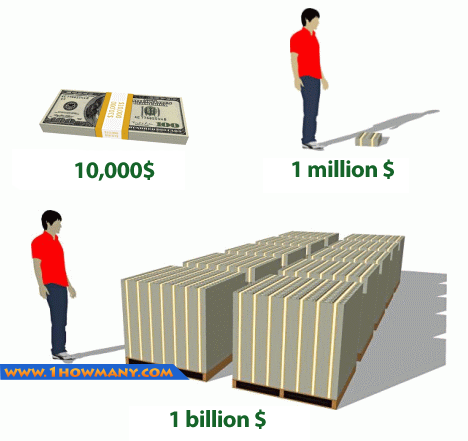﻿ How Many Zeroes Are in a Billion
www.1howmany.com
Units of Measure / How Many Zeroes Are in a Billion

# How Many Zeroes Are in a Billion

The question, “How many zeroes are in a billion?” may seem ridiculous. But are you sure that you know the exact answer to it? If you ask Americans or Europeans about it, most probably, they will tell you the following:

• There are nine zeroes in one billion

But citizens of many non-English speaking countries may give you another answer:

• There are twelve zeroes in one billion

What answer is right? If fact, both variants are, and now you will know why it is so.

The Long Scale and the Short Scale

In the modern world, there are two different scales, which use the term “billion”. They are called the long one and the short one. These terms were first used by Genevieve Guitel, the French mathematician.

In the short scale1, a billion consists of a thousand millions. It means that it has nine zeroes. But in the long scale2, a billion means a million millions. Thus, it has twelve zeroes.

Short Scale
Long Scale
A million
1 000 000
1 000 000
A billion
1 000 000 000
1 000 000 000 000
A trillion
1 000 000 000 000
1 000 000 000 000 000 000

In some countries the word “milliard” is used to denote the nine zero number.

As a rule, in English speaking countries the short scale is in use, while most of other countries prefer the long scale.

It is not easy for an ordinary man to comprehend the practical meaning of a billion. Read these facts, and you may be amazed.

• One billion minutes ago Jesus Christ was alive.
• One billion hours ago it was the Stone Age.
• One billion days ago there were no men on the Earth.

Do you understand now how rich Bill Gates is? He’s got tens of billions dollars.1. The short scale (from French échelle courte) is a system of large number naming. According to the short scale, starting from million, every number is one thousand times the previous number. E.g. billion is a thousand millions.
2. The long scale (from French échelle longue) is a system of large number naming. According to the long scale, starting from million, every number is one million times the previous number. E.g. billion is a million millions.
You may be interested in: